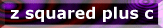Index Page

Gallery 1

Gallery 2

Gallery 3

Gallery 4

Gallery 5

Colouring methods:
page 1
page 2

Image info

Request page# Implementing Colouring Methods

Lets start with a simple example using the Fractint formula parser (presuming you have some knowledge of this). First, the standard Mandelbrot:

```NormalMandel {
z=0, c=pixel:

z=z*z+c

|z| <= 4
}
```

We are going to use the outside=real option on the 'x' screen to implement the colouring methods. This option returns iter+real(zb) where zb is the bailout value of z. What we have to do is to mess around with real(zb) without altering the orbit of z. What I do is to use a flag, that checks whether the bailout condition has been reached. If it has, then the value of z can be changed, as we have reached the end of the iteration. In the following example, we are simply going to set real(zb) to double what it should be

```MandelCol1 { ;
z=0, c=pixel, flag=0:

z=z*z+c

IF (|z| <= 4)
z=z*2
flag=1
END IF

flag==0
}
```

Remember that the formula is iterated until the last statement returns a false value, this is why you have the flag==0 line. If we want to extend this to having a running average/sum whatever, we need at least another counter. For one of my trap methods, we need an IF..END IF to see whether a certain z is within a trap, and to colour it, if it is. The equtaion of a circle, centre b, radius a, on the z plane is:

| z - b | = a

In Fractint's non-standard notation, this becomes:
cabs(z - b) = a

All points within the circle are given by:
cabs(z - b) < a

and the distance of a point from the edge of circle is given by:
a - cabs(z-b)

We also need something to multiply the colouring to any amount we want - I call it the colour factor. So, the formula we could use to implement the fractal seen on the previous page, would be something like this:

```MandelCircle1 { ;
z=0, c=pixel, flag=0
b=p1, a = real(p2), col=imag(p2), q=0:

z=z*z+c

IF (cabs(z-b) < a)
q=q+(a - cabs(z-b))
END IF

IF (|z| <= 4)
z=q*col
flag=1
END IF
flag==0
}
```

This won't give you quite what you want, because Fractint is still adding the number of iterations to real(zb). Another problem is, that if iter+real(zb) is larger than maxiter, Fractint thinks the point is an inside point. The following formula fixes both these problems, adds a small speed-up (using the test variable), doesn't bother with the a, b and col, and uses real(p3) for the bailout:

```MandelCircle1 { ;
z=0, c=pixel, flag=0, q=0, count=0:

z=z*z+c
count=count+1

test=real(p2)-cabs(z-p1)
IF (test < 0)
q=q+test
END IF

IF (|z| > real(p3))
q=q*imag(p2)
z=q-255*floor((q-1)/real(255))
z=z-count
flag=1
END IF

flag==0
}
```

Explanation of the floor function bit: Ideally, whenever q becomes greater than 255, we want it wrapped around to the beginning. Personally, I use colour zero for inisde colour, and colours 1 to 255 for outside, allowing me to cycle 1 to 255. Colour 256 should therefore be mapped to colour 1, 257 to 2 etc. The floor function rounds down to the nearest integer, so that a multiple of 255 is always removed from q, ie a palettes worth. The number of iterations is then subtracted by the formula, and Fractint will just add it straight back on.

For more extravagant shapes, it may be easier to just ignore the complex number bit and consider the separate components. Also, remember that there are many other variations on my methods - you don't have to sum all the values, maybe just the last one, maybe the first, maybe a weighted average, maybe those... Completely different traps are possible, that don't invovle shapes so much as angles, distances of approach etc. I hope this tutorial has helped you unleash more out of Fractint's formula parser. If you want them, you can download my formula files from the download page.colouring methods